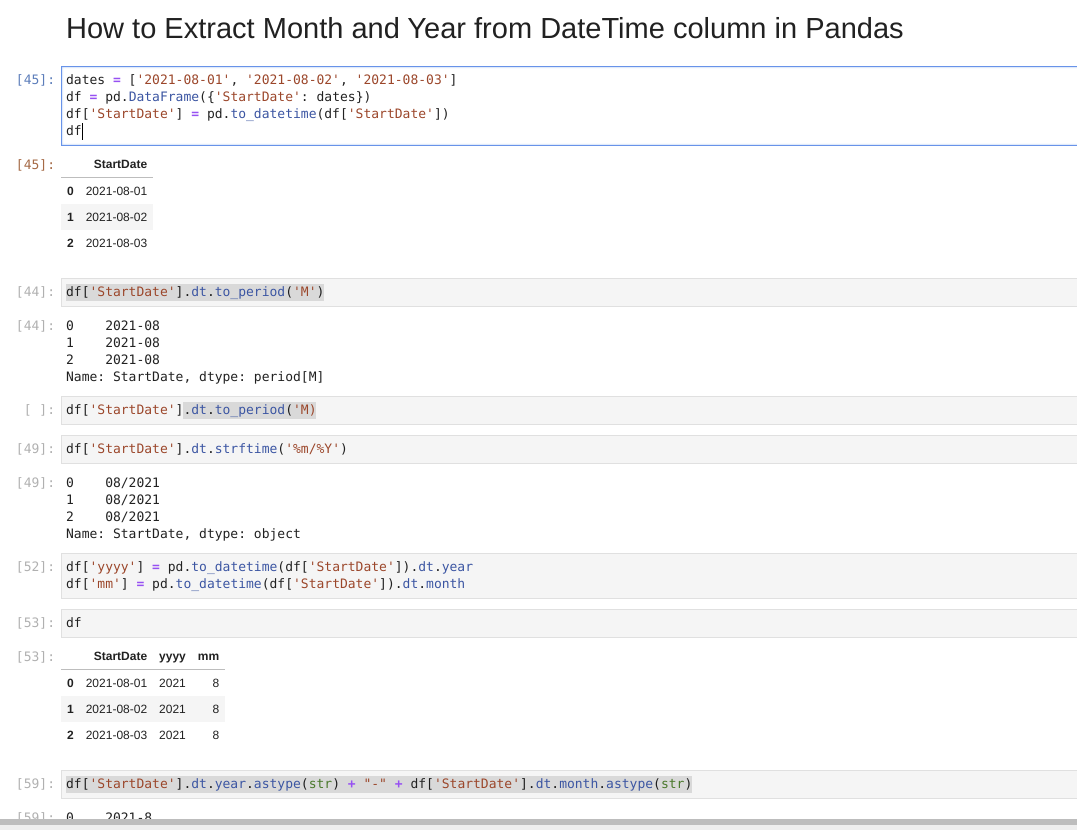In this short guide, I'll show you how to extract Month and Year from a DateTime column in Pandas DataFrame. You can also find how to convert string data to a DateTime. So at the end you will get:

`01/08/2021` -> `2021-08`
`DD/MM/YYYY` -> `YYYY-MM`

or any other date format. We will also cover `MM/YYYY`.

To start, here is the syntax that you may apply in order extract concatenation of year and month:

``````.dt.to_period('M')
``````

In the next section, I'll review the steps to apply the above syntax in practice.

## Step 1: Create a DataFrame with Datetime values

Lets create a DataFrame which has a single column StartDate:

``````dates = ['2021-08-01', '2021-08-02', '2021-08-03']
df = pd.DataFrame({'StartDate': dates})
``````

result:

StartDate
2021-08-01
2021-08-02
2021-08-03

In order to convert string to Datetime column we are going to use:

``````df['StartDate'] = pd.to_datetime(df['StartDate'])
``````

## Step 2: Extract Year and Month with .dt.to_period('M') - format YYYY-MM

In order to extract from a full date only the year plus the month: 2021-08-01 -> 2021-08 we need just this line:

``````df['StartDate'].dt.to_period('M')
``````

result:

``````0    2021-08
1    2021-08
2    2021-08
``````

## Step 3: Extract Year and Month other formats MM/YYYY

What if you like to get the month first and then the year? In this case we will use `.dt.strftime` in order to produce a column with format: `MM/YYYY` or any other format.

``````df['StartDate'].dt.strftime('%m/%Y')
``````
``````0    08/2021
1    08/2021
2    08/2021
``````

Note: have in mind that this solution might be really slow in case of a huge DataFrame.

## Step 4: Extracting Year and Month separately and combine them

A bit faster solution than step 3 plus a trace of the month and year info will be:

• extract month and date to separate columns
• combine both columns into a single one
``````df['yyyy'] = pd.to_datetime(df['StartDate']).dt.year
df['mm'] = pd.to_datetime(df['StartDate']).dt.month
``````
StartDate yyyy mm
2021-08-01 2021 8
2021-08-02 2021 8
2021-08-03 2021 8

and then:

``````df['yyyy'].astype(str) + '-'+ df['mm'].astype(str)
``````

Note: If you don't need extra columns you can just do:

``````df['StartDate'].dt.year.astype(str) + "-" + df['StartDate'].dt.month.astype(str)
``````

Notebook with all examples: Extract Month and Year from DateTime column